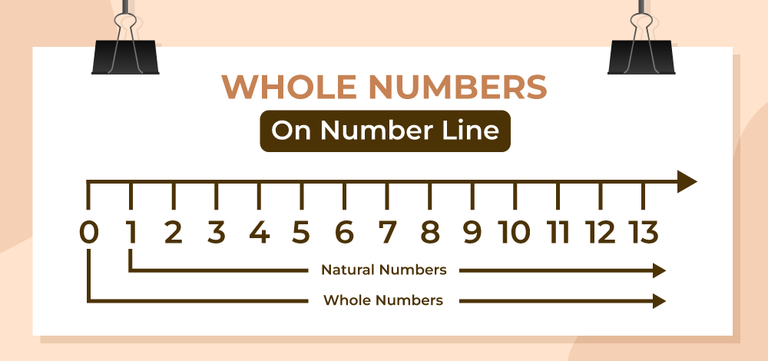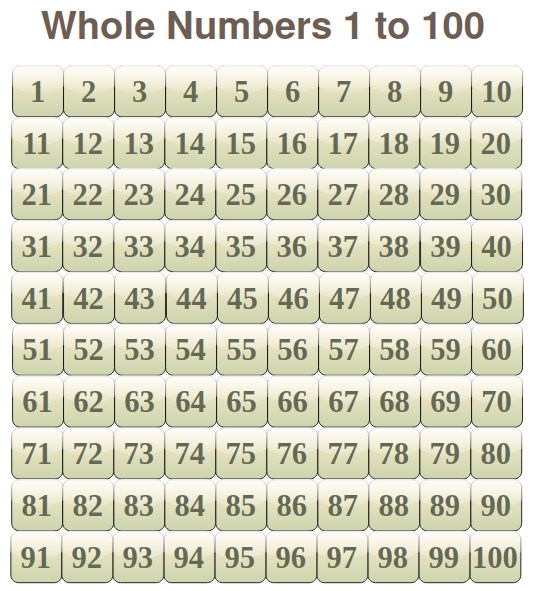# How many whole numbers are there between 1 and 100?

Whole numbers is a group of natural numbers or counting numbers including the number zero. All the numbers in the range from 1 to 100 are Whole numbers. There are a total of 100 numbers between 1 to 100.

## Whole Number

The counting numbers including 0 are known as whole numbers. We know that the numbers 1, 2, 3, 4, 5, … etc. are the counting numbers, and the numbers 0, 1, 2, 3, 4, 5, 6, … etc are the whole numbers. Whole numbers are denoted as W and Natural numbers are denoted as N. Therefore, we can represent whole numbers as,

• W = {0, 1, 2, 3, 4, 5, ….}
• W= {N} + 0

We can easily represent the whole numbers on the number line and the on the right side of 0 (including 0) in the number line are whole numbers.

The image added below shows the whole number on the number line.## List of Whole Numbers from 1 to 100

All the numbers from 0 to infinity are whole numbers, while counting whole numbers between 1 and 100, no numbers are left out and all the numbers are considered whole numbers. There are a total of 100 whole numbers from 1 to 100. In this list, the smallest whole number is 1 and the largest whole number is 100.

The list containing all the numbers from 1 to 100 is,## Sum of Whole Numbers from 1 to 100

We know that whole numbers from 1 to 100 are, 1, 2, 3, 4, 5, ….., 100. All the numbers in this list are in AP and their common difference is 1 and the first and the last term are 1 and 100 respectively. Using all this information we can easily get the sum of all the whole numbers from 1 to 100. For calculating the sum of,

S = 1+2+3+4+5+…..+100

First Term (a) = 1

Common Diference (d) = 1

Last Term (l) = l

Using the sum of the AP formula,

S = n/2[a + l]

S = 100/2 [1 + 100]

S = 50

S = 5050

Thus, the sum of whole numbers from 1 to 100 is 5050.

## Average of Whole Numbers from 1 to 100

Now after finding the sum of the whole numbers from 1 to 100, we can easily find the average of the whole numbers from 1 to 100 using the average formula as,

Average = (Sum of Terms)/(Number of Terms)

From the above heading, we know that the sum of whole numbers from 1 to 100 is 5050.

The number of whole numbers from 1 to 100 is 100.

Now using the average formula,

A = 5050/100

A = 50.5

Thus, the average of all whole numbers from 1 to 100 is 50.5

## Operations on Whole Numbers

We can perform various operations on the whole number including,

• Subtraction of Whole Number
• Multiplication of Whole Number
• Division of Whole Number

Now let’s learn about them in detail.

We can simply perform addition on whole numbers and the addition of a whole number represents a whole number. This can be better understood with the example discussed below,

Example: Find the sum of 27 + 11

Solution:

Given,

= 27 + 11

As both the numbers are whole numbers their addition is also a whole number. Finding their sum.

= 38 (whole number)

This is the required sum.

### Subtraction of Whole Number

We can simply perform subtraction on whole numbers and the subtraction of a whole number may or may not represents a whole number. This can be better understood with the example discussed below,

Example: Find the difference, between 27 – 11 and 11 – 27

Solution:

Given,

= 27 – 11

As both the numbers are whole numbers their subtraction may or may not results in a whole number.

= 16 (whole number)

This is the required difference.

Given,

= 11 – 27

= -16 (Integer)

This is the required difference.

### Multiplication of Whole Number

We can simply perform multiplication on whole numbers and the multiplication of a whole number represents a whole number. This can be better understood with the example discussed below,

Example: Find the product of 27 × 11

Solution:

Given,

= 27 × 11

As both the numbers are whole numbers their multiplication is also a whole number. Finding their product.

= 297 (whole number)

This is the required product.

### Division of Whole Number

We can simply perform division on whole numbers and the division of a whole number may or may not result in a whole number. This can be better understood with the example discussed below,

Example: Solve

• 27 / 11
• 27 / 9

Solution:

Given,

= 27 / 11

Dividing them,

= 2.4545 (Decimal)

Now,

Given,

= 27 / 9

Dividing them,

= 3 (Whole Number)

## Solved Examples on Whole Number

Example 1: Find the sum of 34 + 45

Solution:

Given,

= 34 + 45

As both the numbers are whole numbers their addition is also a whole number. Finding their sum.

= 79 (whole number)

This is the required sum.

Example 2: Find the difference 89 – 67.

Solution:

Given,

= 89 – 67

= 23 (whole number)

This is the required difference.

Example 3: Find the product of 7 × 3

Solution:

Given,

= 7 × 3

As both the numbers are whole numbers their multiplication is also a whole number. Finding their product.

= 21 (whole number)

This is the required product.

Example 4: Solve, 48 / 4

Solution:

Given,

= 48 / 4

Dividing them,

= 12 (Whole Number)

## FAQs

### Q1: What are Whole Numbers?

The group of numbers containing all the natural numbers including the Zero(0) is called the whole numbers. It is represented using the symbol W. Examples of whole numbers are, 0, 3, 89, etc.

### Q2: Is 0 a Whole Number?

Yes, Zero (0) is a whole number.

### Q3: What is the sum of two Whole Numbers?

The sum of two numbers is always a whole number. For example,

• 3 + 4 = 7 (Whole Number)